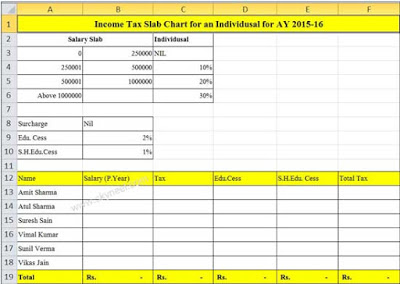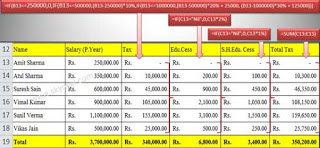# Easy Excel IF Formula for Income-Tax Calculation AY 2015-16

Nested IF function in MS Excel anyone will be able to easily income-tax calculation his yearly Income-Tax amount for any Individua.

Hello friends at present time there are lot of direct and indirect tax charge by the Government. income-tax calculation is an important part for all business or salaried persons. These taxes is used to build strong structure of our economic of our country. Today we have to discuss an important topic how to calculate income tax with the help of IF function in MS Excel. After reading this article Easy Excel IF Formula for Income-Tax Calculation AY 2015-16 you will be able to easily calculate your income tax.

With the help of this article You can easily compute your tax liabilities for salaried individuals persons.  With the help of income-tax calculation in MS Excel you will use Nested IF Function. “IF” and “Nested IF” functions are very popular functions in “Logical functions” category in MS Excel. Nested IF function is used when another IF function is assigned inward the first IF function in order to check extra situations.

### Easy Excel IF Formula for Income-Tax Calculation AY 2015-16:

For example, IF you want to calculate income tax, education cess and secondary higher education-cess as per current slab for income tax of AY 2015-16 for the Individual, the slab rate of income tax is given below:

Individual resident whose age is lower than sixty years (That means whose date of birth is on or after the 1st Apr. , 1953) or any Non Resident Indian / Hindu UN-Divided Family / Association of Person / Body of Individuals / Artificial Judicial Person

#### Income-tax: Calculate Liability for Tax for AY 2015-16

 S. No. Income Slabs Income Tax Rate 1. Where the total earning is not greater than equal to Rs. 2,50,000/-. – 2. Where the total earning is greater than Rs. 2,50,000/- but less than Rs. (500000) five lakhs. 10% of the sum of earning which greater than Rs. 2,50,000/- 3. Where the total earning is greater than Rs. (5,00,000) lakhs but less than Rs. (1000000) lakhs. Rs. (25,000) Twenty Five Thousand + 20% of the whole amount which is the whole earning larger than Rs. (500000) five lakhs/-. 4. Where the total income greater than Rs. (10,00,000) Ten Lakhs. Rs. (1,25,000) One Lakh and Twenty Five Thousand + 30% of the whole amount which is the whole earning larger than Rs. (1000000) Ten Lakhs.

Education Cess: 2% of the Income Tax Amount.
Secondary Higher Education Cess: 1% of the Income Tax Amount.
Steps to calculate Income tax as for Individual for AY 2015-16:
If you want to calculate income tax for any Individual for the Assessment Year 2015-16 as per the given table with the help of “Nested-IF” function in MS Excel, then you have to take following few important steps.

Step 1: Prepare the following given sheet in MS Excel as per your need for income-tax calculation.Step 2: If you want to calculate income tax amount then you have to type given formula in the cell C13:
=IF(B13<=250000,0,IF(B13<=500000,(B13-250000)*10%,IF(B13<=1000000,(B13-500000)*20% + 25000, (B13-1000000)*30% + 125000)))

Step 3: After calculating the income tax amount now, if you want to calculate education cess which is applicable only on income tax amount then you have to type given formula to calculate education cess @ 2% on income tax in cell D13:
=IF(C13=”Nil”,0,C13*2%)

Step 4: Now, you have to calculate S.H.Edu.Cess amount which is also calculated on the basis on income tax amount so, you have to type following given formula to calculate S.H.Education Cess @ 1% on income tax in cell E13:
=IF(C13=”Nil”,0,C13*1%)

Step 5: Now, to calculate total tax amount then you have to type following formula in cell F13:
=SUM(C13:E13)

Step 6: Copy formula of Income tax, Edu. Cess and S.H.Edu.Cess by drag and drop method in given range C13:F18.Step 7: Finally you see income-tax calculation process and compute total tax amount as per given income tax slab of AY 2015-16.

I hope you like this post. This post is useful to  income-tax calculation only for individuals every year, but you have to change income tax slab as per given income tax department. Give your important suggestion about income-tax calculation in the comment box.and also share this article with your friends to show your knowledge in excel. Thanks.